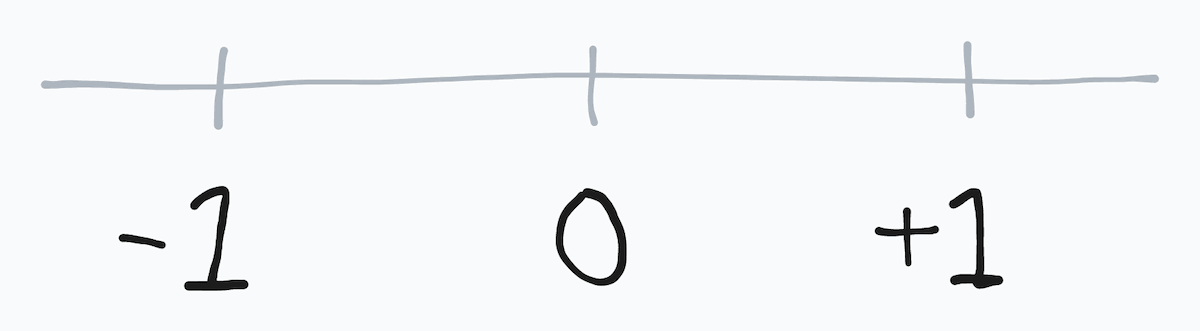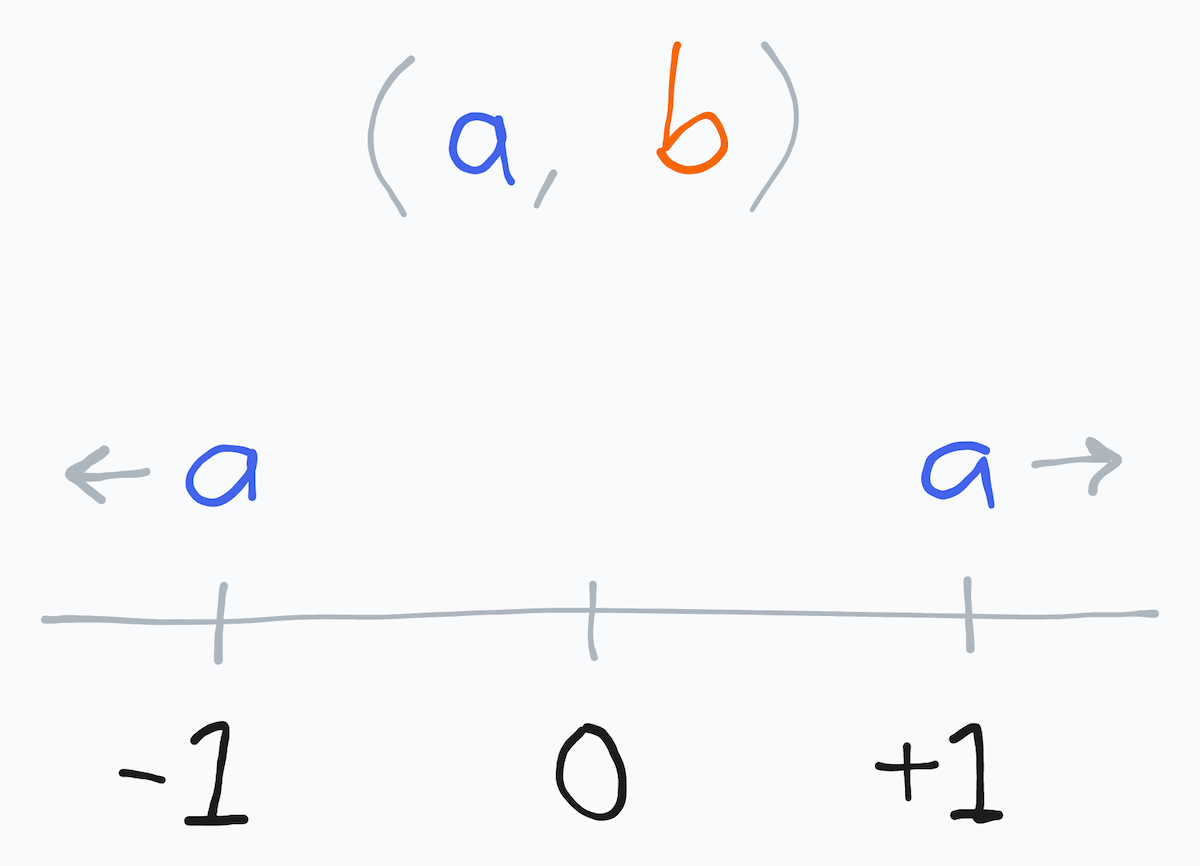# Visual mnemonic for an array sorter's return values

Published on in JavaScript and Mnemonics

When sorting an array with a custom comparator function, the function should return `<0`, `0` or `>0`. But what do the values do? Use this weird visual mnemonic – sorting consultants HATE it!

First, recall that an array sorter compares two values, `a` and `b`:

``````array.sort((a, b) => {
// ...
})
``````

Second, because the sorter function should return a number, imagine an x-axis with the numbers `-1`, `0` and `+1`:Now, realize that `-1` is on the left side of the x-axis, and `+1` is on the right side:

• Returning `-1` (or anything less than zero) from the array sorter moves `a` to the left (before `b` in the array).
• Returning `+1` (or anything greater than zero) from the array sorter moves `a` to the right (after `b` in the array).
• (Returning `0` keeps the order of `a` and `b`.)

And why is `a` moved relative to `b` – because the array sorter compares two values (`a` and `b`), and `a` comes first in the function parameters (`(a, b) => {}`). First come, first served.

Like so:That's it! To recap:

``````array.sort((a, b) => {
return -1 // <-a
return +1 //   a->
return 0 // Keep order of `a` and `b`
})
``````

(Illustrations mouse-drawn on tldraw.com.)